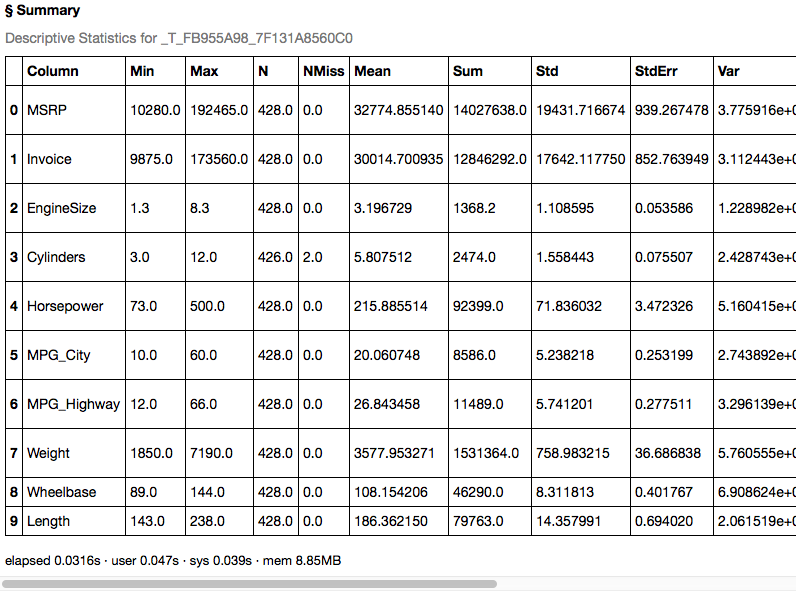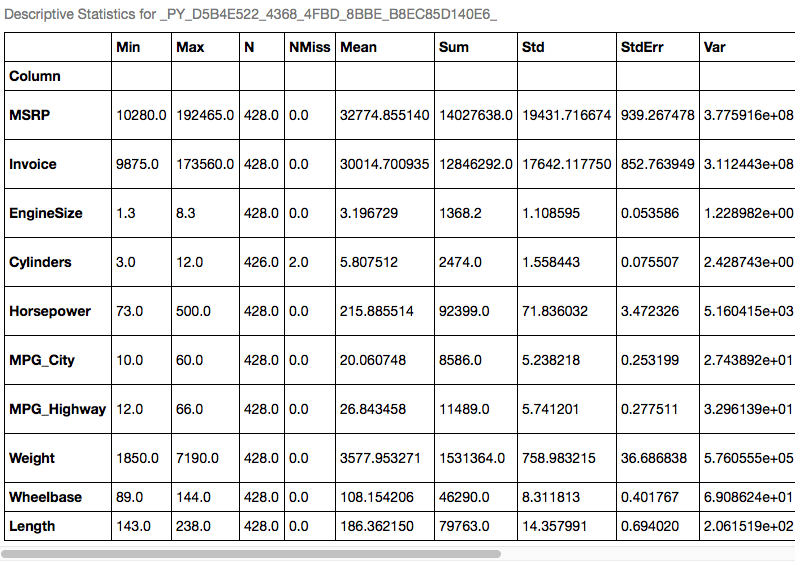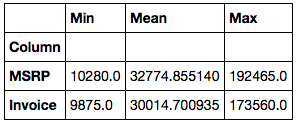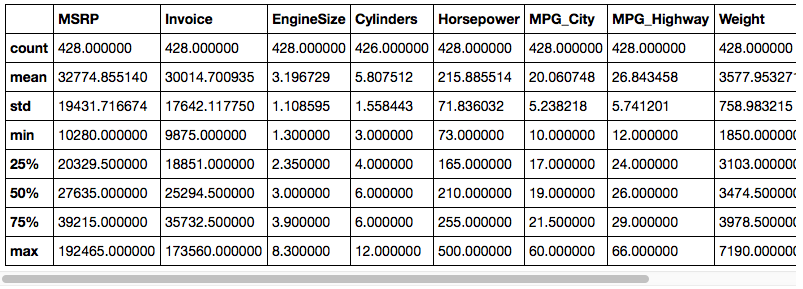## Simple Statistics in Python

Started ‎04-13-2016 by
Modified ‎04-16-2019 by
Views 608

# Simple Statistics in Python

The actions in CAS cover a wide variety of statistical analyses. While we can't cover all of them here, we'll at least get you started on some of the simpler ones.

First we need to get a CAS connection set up.

``````In: import swat

## The `simple` Action Set

The basic statistics package in CAS is called simple and should be already loaded. If you are using IPython, you can see what actions are available using the operator.

``````In: conn.simple?
Type:        Simple
String form: <swat.cas.actions.Simple object at 0x3cf0d90>
File:        /u/kesmit/pp/swat/GTKLAXND/misc/python/swat/cas/actions.py
Definition:  conn.simple(self, *args, **kwargs)
Docstring:
Analytics

Actions
-------
simple.correlation : Generates a matrix of Pearson product-moment correlation coefficients
simple.crosstab    : Performs one-way or two-way tabulations
simple.distinct    : Computes the distinct number of values of the variables in the variable list
simple.freq        : Generates a frequency distribution for one or more variables
simple.groupby     : Builds BY groups in terms of the variable value combinations given the variables in the variable list
simple.mdsummary   : Calculates multidimensional summaries of numeric variables
simple.numrows     : Shows the number of rows in a Cloud Analytic Services table
simple.paracoord   : Generates a parallel coordinates plot of the variables in the variable list
simple.regression  : Performs a linear regression up to 3rd-order polynomials
simple.summary     : Generates descriptive statistics of numeric variables such as the sample mean, sample variance, sample size, sum of squares, and so on
simple.topk        : Returns the top-K and bottom-K distinct values of each variable included in the variable list based on a user-specified ranking order``````

You can also use Python's help function.

``````In: help(conn.simple)
Help on Simple in module swat.cas.actions object:

class Simple(CASActionSet)
|  Analytics
|
|  Actions
|  -------
|  simple.correlation : Generates a matrix of Pearson product-moment correlation coefficients
|  simple.crosstab    : Performs one-way or two-way tabulations
|  simple.distinct    : Computes the distinct number of values of the variables in the variable list
|  simple.freq        : Generates a frequency distribution for one or more variables
|  simple.groupby     : Builds BY groups in terms of the variable value combinations given the variables in the variable list
|  simple.mdsummary   : Calculates multidimensional summaries of numeric variables
|  simple.numrows     : Shows the number of rows in a Cloud Analytic Services table
|  simple.paracoord   : Generates a parallel coordinates plot of the variables in the variable list
|  simple.regression  : Performs a linear regression up to 3rd-order polynomials
|  simple.summary     : Generates descriptive statistics of numeric variables such as the sample mean, sample variance, sample size, sum of squares, and so on
|  simple.topk        : Returns the top-K and bottom-K distinct values of each variable included in the variable list based on a user-specified ranking order
|
|  Method resolution order:
|      Simple
|      CASActionSet
|      builtins.object
|
|  Data and other attributes defined here:
|
|  actions = {'correlation': <class 'swat.cas.actions.simple.Correlation'...
|
|  ----------------------------------------------------------------------
|  Methods inherited from CASActionSet:

... output truncated ...``````

Let's start off with the summary action. We'll need some data, so we'll load some CSV from a local file. Then we'll run the action on it.

``````In: cars = conn.read_csv('https://raw.githubusercontent.com/sassoftware/sas-viya-programming/master/data/cars.csv')
In: out = cars.summary()
In: out``````The result object here is a CASResults object which is a subclass of a Python dictionary. In this case, we only have one key "Summary". The value for this key is a DataFrame. We can store the DataFrame in a variable so that it's easier to work with, then we can do any of the standard Pandas DataFrame operations on it. Here we are setting the first column as the index for the DataFrame so that we can do data selection easier later on.

``````In: df = out['Summary']
In: df.set_index(df.columns, inplace=True)
In: df``````Now that we have an index, we can use the loc property of the DataFrame to select rows based on index values as well as columns based on names.

``In: df.loc[['MSRP', 'Invoice'], ['Min', 'Mean', 'Max']]``## DataFrame methods on CASTable objects

In the previous example, we called the summary action directly. This gave us a CASResults object that contained a DataFrame with the result of the action. You can also use many of the Pandas DataFrame methods directly on the CASTable object so that, in many ways, they are interchangeable. One of the most common methods used on a Pandas DataFrame is the describe method. This includes statistics that would normally be gotten by running variations of the summarydistincttopk, and percentile actions. This is all done for you and the output created is the same as what you would get from an actual Pandas DataFrame. The difference is that in the case of the CASTable version, you can handle much, much larger data sets.

``In: cars.describe()``Other examples of DataFrame methods that work on CASTable objects are minmaxstd, etc. Each of these calls summary in the background, so if you want to use more than one, you might be better off just calling the describe method once to get all of them.

``````In: cars.min()
Out:
Make                Acura
Model          3.5 RL 4dr
Type               Hybrid
Origin               Asia
DriveTrain            All
MSRP                10280
Invoice              9875
EngineSize            1.3
Cylinders               3
Horsepower             73
MPG_City               10
MPG_Highway            12
Weight               1850
Wheelbase              89
Length                143
dtype: object

In: cars.max()
Out:
Make                             Volvo
Model          Z4 convertible 3.0i 2dr
Type                             Wagon
Origin                             USA
DriveTrain                        Rear
MSRP                            192465
Invoice                         173560
EngineSize                         8.3
Cylinders                           12
Horsepower                         500
MPG_City                            60
MPG_Highway                         66
Weight                            7190
Wheelbase                          144
Length                             238
dtype: object

In: cars.std()
Out:
MSRP           19431.716674
Invoice        17642.117750
EngineSize         1.108595
Cylinders          1.558443
Horsepower        71.836032
MPG_City           5.238218
MPG_Highway        5.741201
Weight           758.983215
Wheelbase          8.311813
Length            14.357991
dtype: float64
``````

## Conclusion

Although we have just barely scratched the surface, you should now be able to get some basic statistical results back about your data. Whether you want to use the action API directly, or the familiar Pandas DataFrame methods is up to you.

## Resources